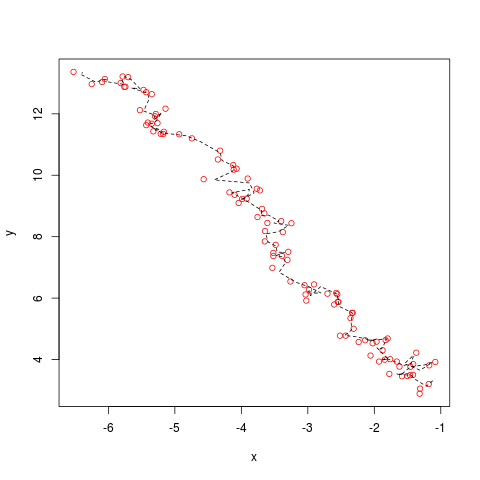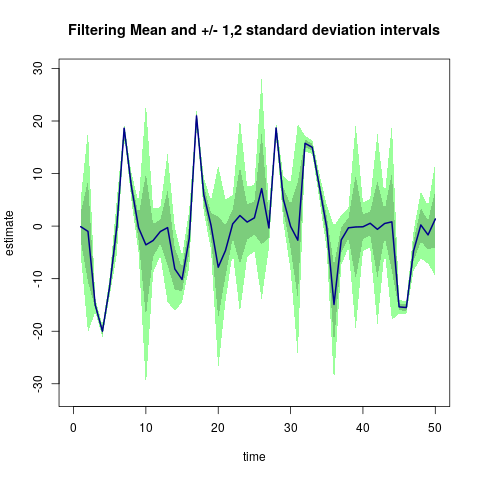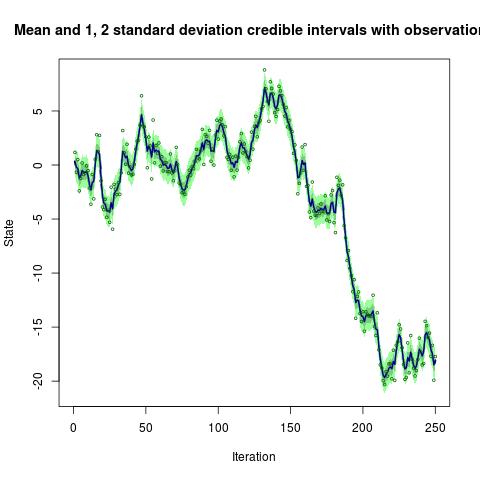## RcppSMC: Sequential Monte Carlo and Particle Filters via Rcpp

### Overview

RcppSMC combines SMCTC template classes for Sequential Monte Carlo and Particle Filters (Johansen, 2009, J Statistical Software, 30:6) with the Rcpp package for R/C++ Integration (Eddelbuettel and Francois, 2011, J Statistical Software, 40:8). RcppSMC allows for easier and more direct access from R to the computational core of the SMC algorithm.

Sequential Monte Carlo methods are a very general class of Monte Carlo methods for sampling from sequences of distributions. Simple examples of these algorithms are used very widely in the tracking and signal processing literature. Recent developments illustrate that these techniques have much more general applicability, and can be applied very effectively to statistical inference problems. Unfortunately, these methods are often perceived as being computationally expensive and difficult to implement.

By combining the SMCTC with the ‘glue’ provided by Rcpp, a tighter integration with R is achieved. This allows the applied researcher interested in Sequential Monte Carlo and Particle Filter methods to more easily vary input data, summarize outputs, plot results and so on.

As a concrete example, figure 5.1 of Johansen (2009) which illustrates a Particle Filter for a two-dimensional linear state space model with non-Gaussian observation error, is reproduced by

``res <- pfLineartBS(plot=TRUE)``

where we select the optional plot. Moreover, progress during the model fit can also be visualized (using callbacks into R from C++ which Rcpp provides) via

``res <- pfLineartBS(onlinePlot=pfLineartBSOnlinePlot)``

where `pfLineartBSOnlinePlot()` is a default plotting function provided for this example by the package.

Two more ‘classic’ examples from the literature have been added to the package:

• `blockpfGaussianOpt()` provides the Block Sampling Particle Filter of Doucet, Briers and Senecal (2006, JCGS 15:693) in the context of a univariate linear Gaussian model.
• `pfNonlinBS()` provides the Bootstrap Particle Filter of Gordon, Salmond and Smith (1993, IEE Proceedings-F 140:107) in the context of the ubiquitous nonlinear model which was used in section 4.1 of that paper.

These examples are hopefully of some pedagogic interest and provide templates which can be used to guide the implementation of more complicated algorithms using the Rcpp/SMCTC-combination.

We intend to add more example and illustrations over time and aim ultimately to provide a framework to support the straightforward implementation of SMC algorithms which exploits the powerful combination of R and C++.

### So give me an example!

As of version 0.1.0, the functions are included which reproduce examples from the literature. As noted above, running

``res <- pfLineartBS(plot=TRUE)``

(which is part of `example(pfLineartBS)` too) creates the following chartRunning the example for the function `pfNonlinBS()`, that is

``````sim <- simNonlin(len=50)
res <- pfNonlinBS(sim\$data,particles=500,plot=TRUE)``````

creates the following plot (which reproduces example 4.1 of Gordon, Salmon and Smith (1993) and their nonlinear model)Note that the data is freshly simulated, so the chart will vary unless the RNG seed is fixed. Lastly, running

``````sim <- simGaussian(len=250)
res <- blockpfGaussianOpt(sim\$data,lag=5,plot=TRUE)``````

reproduces the example from Doucet, Briers and Senecal (2006):### Where do I get it?

RcppSMC is now a CRAN package. Its source can be found on GitHub within the rcppsmc organization. More information about SMCTC is at this page.

### Authors

RcppSMC is being written by Dirk Eddelbuettel, Adam M. Johansen and Leah F. South.

### License

RcppSMC is licensed under the GNU GPL version 2 or later.

Initially created: Wed Jan 11 21:19:04 CST 2012
Last modified: Sun Jul 19 18:55:43 CDT 2020